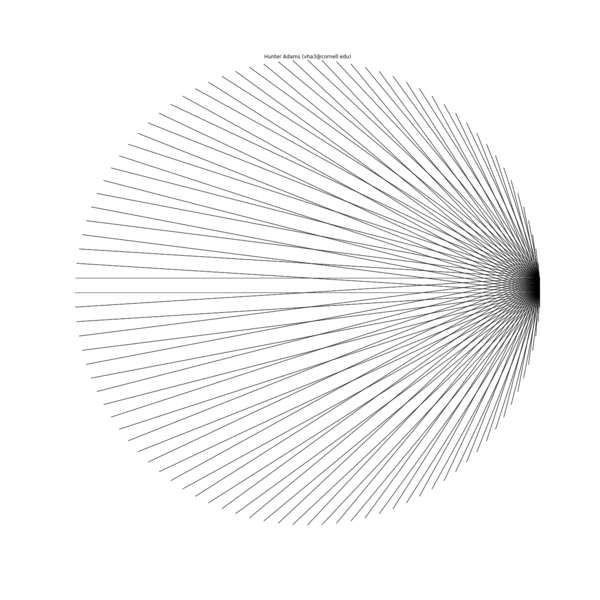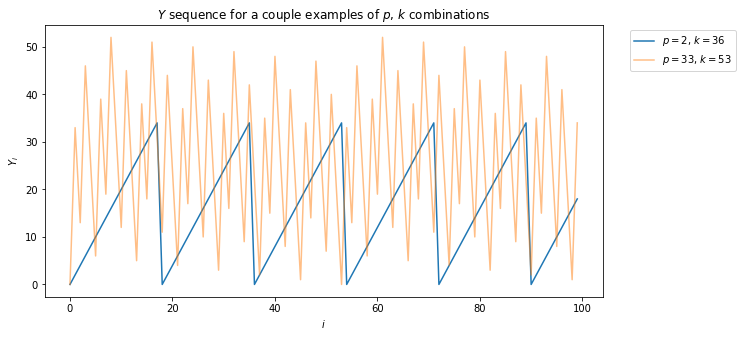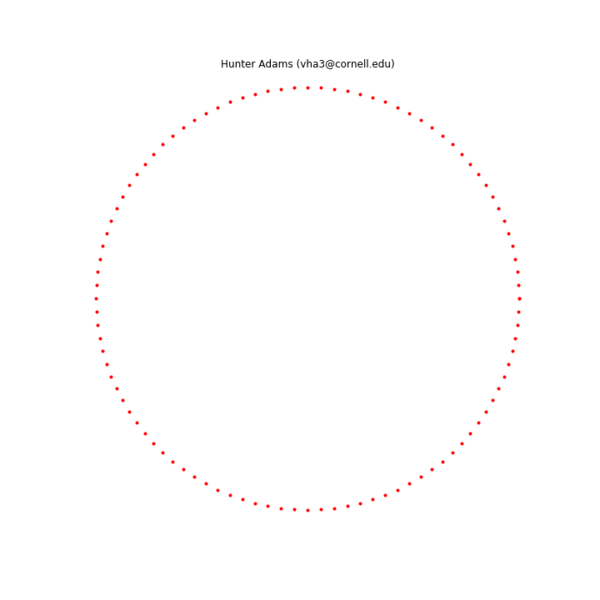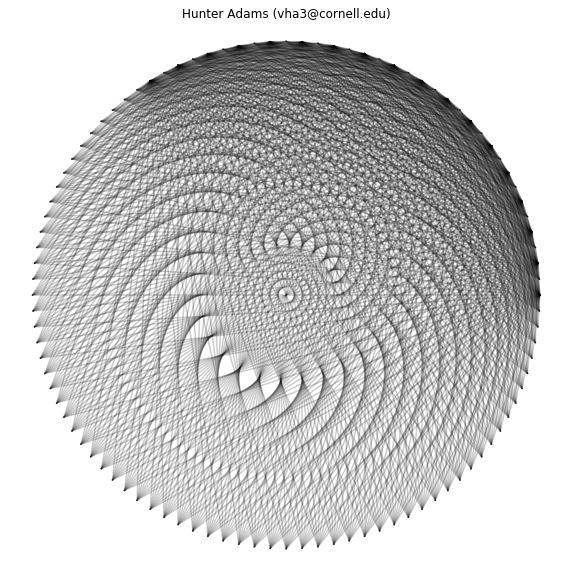# Attempting to understand something beautiful¶

In :
import plotly.graph_objects as go
import numpy
import matplotlib.pyplot as plt
from scipy.interpolate import InterpolatedUnivariateSpline
from scipy.integrate import odeint
from IPython.display import Latex
from IPython.display import Image
import networkx as nx
import csv
import binascii
%matplotlib inline
plt.rcParams['figure.figsize'] = [10, 10]
from IPython.core.display import HTML
HTML("""
<style>
.output_png {
display: table-cell;
text-align: center;
vertical-align: middle;
}
</style>
""")

Out:

## On beautiful things¶

In playing with simulations of simple rules for stringing wires across circularly distributed pegs, I discovered a family of rules that generated a particularly beautiful set of patterns, shown below. This document is an extended investigation of these string patterns that is an attempt to mine them for the properties that make them beautiful.## Some mysteries to solve in this document:¶

1. Why are some patterns symmetric, and others are asymmetric?
2. Why do some seem to show much more complexity and many more strings than others?
3. Are these forming closed networks? If so, how many?
4. What are the properties of the networks that are being formed?
5. Which of these patterns could be built with a single string?

## What are we looking at?¶

The above plot is a visual representation of the interaction between two integer sequences, which I will call $Y_1$ and $Y_2$.

\begin{align} %X_i &= i\mod{N} \hspace{0.5cm} \text{for $i$}\in[0,1,2,\dots]\\ Y_1 &= \left(p_1 \cdot i\right)\mod{k_1} \hspace{0.5cm} \text{for $i$}\in[0,1,2,\dots]\\ Y_2 &= \left(p_2 \cdot i\right)\mod{k_2} \hspace{0.5cm} \text{for $i$}\in[0,1,2,\dots] \end{align}

The $Y$ sequence:

The $Y$ sequence has two parameters, $p$ and $k$. The $Y$ sequence is the sequence of integer multiples of $p$ (any non-negative integer) modulo $k$ (any positive integer). This sequence looks very different for different combinations of $p$ and $k$, as illustrated below.

In :
x = numpy.arange(0,100)
plt.rcParams['figure.figsize'] = [10, 5]
plt.plot(x, (x*2)%36, label="$p=2$, $k=36$");
plt.plot(x, (x*33)%53, label="$p=33$, $k=53$", alpha=0.5);
plt.legend(bbox_to_anchor=(1.04,1), loc="upper left")
plt.title('$Y$ sequence for a couple examples of $p$, $k$ combinations');
plt.xlabel('$i$');plt.ylabel('$Y_i$');plt.show()Forming patterns from the sequences:

Each element of the two sequences is connected. Starting with $i=0$ and iterating upward, each $Y_{1,i}$ and $Y_{2,i}$ is calculated and a connection is formed between those with the same index. In other words, each row of the below table is a connection.

$i$ $Y_{1,i}$ $Y_{2,i}$
0 $y_{1,0}$ $y_{2,0}$
1 $y_{1,1}$ $y_{2,1}$
2 $y_{1,2}$ $y_{2,2}$
3 $y_{1,3}$ $y_{2,3}$
$\vdots$ $\vdots$ $\vdots$

The specific geometries of the string patterns depend on where in space one decides to place each element of the sequence. To form the patterns shown above, $k_{max}$ pegs are arranged in a circular pattern, where $k_{max}=\text{max}(k_1,k_2)$. Each peg represents a particular element of the $Y_{max}$ array.

Because the value for $k_{min} = \text{min}(k_1,k_2)$ is less than $k_{max}$, the values contained in the $Y_{min}$ sequence will also not exceed $k_{min}$. The physical placement for each element of the $Y_{min}$ sequence is the $y_{min,i}^{th}$ peg as counted from a designated 0 peg, walking in the same direction that the $Y_{max}$ sequence walks. This choice of arrangement overlays the elements of the two sequences and yields that patterns that piqued my interest. However, one may choose any physical arrangement of the elements of the sequences.

Below is an animation showing the construction of one of these patterns by connecting the two sequences ($p_1=2$, $k_1=36$, $p_2=1$, $k_2=100$), the final configuration, an alternate configuration formed by separating the $Y_{max}$ and $Y_{min}$ sequences physically ($Y_{max}$ on the inside, $Y_{min}$ on the outside), and the transformation of the first geometry into the second. A structure is specified by the connections, the placement of the nodes in physical space is arbitrary.

Iterative construction of an example pattern ($p_1=1$, $k_1=100$, $p_2=2$, $k_2=36$), 10 connection per frameFinal example pattern ($p_1=1$, $k_1=100$, $p_2=2$, $k_2=36$)

In :
theta = numpy.linspace(0, 2*numpy.pi, 101)
x = numpy.cos(theta)
y = numpy.sin(theta)
# plt.plot(x,y,'r.')
# plt.plot(x,y,'b*')
plt.axis('equal')
plt.ylim([-1.1,1.1])
plt.xlim([-1.1,1.1])

# for j in range(1,100):
count=0
plt.axis('off')
for i in range(901):
dice1=count%100
dice2=int(2*count)%(36)
if (dice2%4==0):
plt.plot([x[dice1],x[dice2]], [y[dice1],y[dice2]], color='black', alpha=0.2)
else:# (dice2%2==1):
plt.plot([x[dice1],x[dice2]], [y[dice1],y[dice2]], color='black', alpha=0.2)
count += 1
plt.show()
# plt.savefig('./plots/%d'%j)
# plt.close()Alternate geometry for the same example pattern ($p_1=1$, $k_1=100$, $p_2=2$, $k_2=36$)

In :
theta = numpy.linspace(0, 2*numpy.pi, 101)
theta2 = numpy.linspace(0, 2*numpy.pi, 37)
x = 0.5*numpy.cos(theta)
y = 0.5*numpy.sin(theta)
x2=1.1*numpy.cos(theta2)
y2=1.1*numpy.sin(theta2)
# plt.plot(x,y,'b.')
# plt.plot(x,y,'black','.')
plt.axis('equal')
plt.ylim([-1.1,1.1])
plt.xlim([-1.1,1.1])

# for j in range(1,100):
count=0
plt.axis('off')
for i in range(901):
dice1=count%100
dice2=int(2*count)%(36)
if (dice2%4==0):
plt.plot([x[dice1],x2[dice2]], [y[dice1],y2[dice2]], color='black', alpha=1, linewidth=0.1)
else:# (dice2%2==1):
plt.plot([x[dice1],x2[dice2]], [y[dice1],y2[dice2]], color='black', alpha=1, linewidth=0.1)
#     else:
#         plt.plot([x[dice1],x2[dice2]], [y[dice1],y2[dice2]], color='black', alpha=0.2)
count += 1